# Comparing areas of plots of land | Measurement | Pre-Algebra

##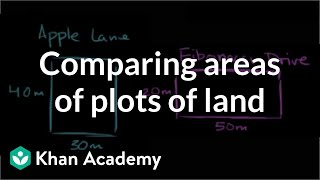By Khan Academy

Find area of two rectangles to solve a word problem.# Area of a Triangle - MathHelp.com - Geometry Help

##By yourteachermathhelp

Students learn the formula for the area of a triangle and are asked to solve problems using this formula. Note that right triangle formulas and trigonometry are used extensively in the problems in this lesson.# Area and perimeter problems - rectangular shapes - 3rd/4th grade math

##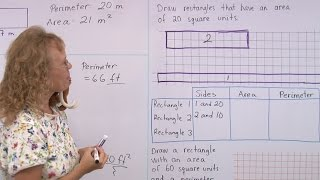By Math Mammoth

After reminding everyone about what is AREA and what is PERIMETER, we solve several problems involving both area and perimeter of rectangles or rectangular shapes.# [3.MD.7b-1.0] Multiply to find Area - Common Core Standard

##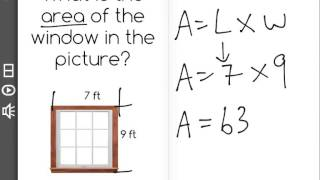By Freckle education

Multiply side lengths to find areas of rectangles with whole-number side lengths in the context of solving real world and mathematical problems# Area of a Trapezoid - YourTeacher.com - Geometry Help

##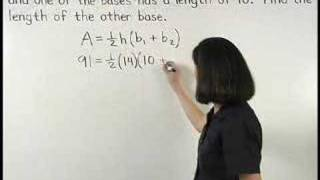By yourteachermathhelp

For a complete lesson on area of a trapezoid go to http://www.yourteacher.com - 1000+ online math lessons featuring a personal math teacher inside every lesson! In this lesson students learn the formula for the area of a trapezoid and are asked to solve problems using this formula. Note that right triangle formulas and trigonometry are used extensively in the problems in this lesson.# Division - The Area Model Word Problems with Single Digit Divisors (4-NBT-B-6)

##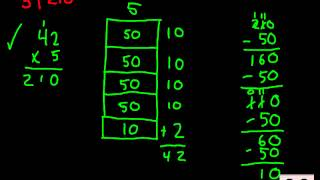By Worksheets and Walkthroughs

This video walkthrough lesson shows you how to use the area model to solve division problems with single digit divisors. Please visit www.worksheetsandwalkthroughs.com to print out the worksheet for this video and others. Also check out our# Area Between Two Curves

##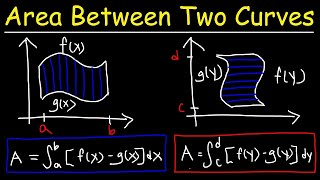By The Organic Chemistry Tutor

This calculus video tutorial provides a basic introduction in finding the area between two curves with respect to y and with respect to x. It explains how to set up the definite integral to calculate the area of the shaded region bounded by the two curves. In order to find the points of intersection, you need to set the two curves equal to each other and solve for x or y. You need to be familiar with some basic integration techniques for this lesson. This video contains plenty of examples and practice problems.# Area of inscribed equilateral triangle (some basic trig used) | Circles | Geometry | Khan Academy

##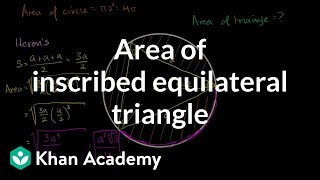By Khan Academy

This video will give you a good feel for what inscribed figures are like. It uses some trigonometry to figure out what the area of an equilateral triangle inscribed in a circle is, given the circle's radius. NOTE: We don't actually need trigonometry to solve this problem. Notice that we can view the equilateral triangle as six identical 30-60-90 triangles, the hypotenuses of which are radii of the circle, then use the 30-60-90 triangle side length ratios to figure out the rest of what we need to know to solve the problem.# Area of inscribed equilateral triangle (some basic trig used) | Circles | Geometry | Khan Academy

##By Khan Academy

This video will give you a good feel for what inscribed figures are like. It uses some trigonometry to figure out what the area of an equilateral triangle inscribed in a circle is, given the circle's radius. NOTE: We don't actually need trigonometry to solve this problem. Notice that we can view the equilateral triangle as six identical 30-60-90 triangles, the hypotenuses of which are radii of the circle, then use the 30-60-90 triangle side length ratios to figure out the rest of what we need to know to solve the problem.# Surface Area and Volume of Spheres - YouTube

##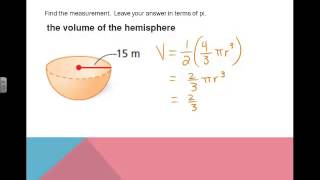By Missy McCarthy

Surface Area and Volume of Spheres - YouTube# Part 2 of proof of Heron's formula | Perimeter, area, and volume | Geometry | Khan Academy

##By Khan Academy

Video shows that the expression in part 1 is identical to Heron's Formula. This video demonstrates how to use Heron's Formula to determine the area of a triangle while only knowing the lengths of the sides# Part 1 of proof of Heron's formula | Perimeter, area, and volume | Geometry | Khan Academy

##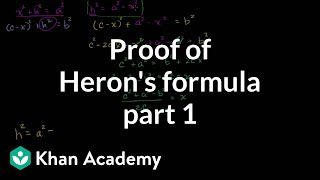By Khan Academy

In this video, the insructor explores and demonsrates the proof of Heron's Formula. The instructor demonstrates with computer software.# Heron's formula | Perimeter, area, and volume | Geometry | Khan Academy

##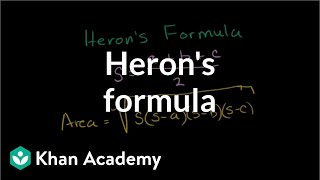By Khan Academy

Using Heron's Formula to determine the area of a triangle while only knowing the lengths of the sides. All Khan Academy content is available for free at www.khanacademy.org# Area of an equilateral triangle | Perimeter, area, and volume | Geometry | Khan Academy

##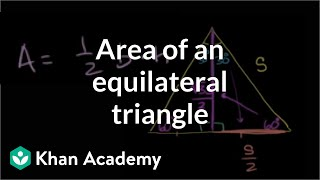By Khan Academy

Finding the formula for the area of an equilateral triangle with side s. All Khan Academy content is available for free at www.khanacademy.org# Inverse Variation | MathHelp.com

##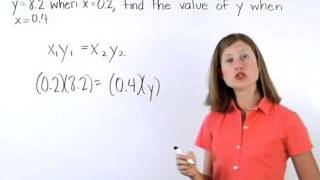By MathHelp.com

This lesson covers the surface area of a pyramid. Students learn the definition of a prism, as well as the following vocabulary words related to prisms: base, base edge, lateral edge, lateral face, and altitude (height). Students also learn the formulas for the lateral area, total area, and volume of a right prism, and are asked to solve problems using these formulas. Note that the prisms in this lesson are primarily rectangular prisms -- prisms whose bases are rectangles.# The surface area and the volume of pyramids, prisms, cylinders and cones

##By MathPlanetVideos

Find the surface area of a cylinder with the radius 4 and height 8# Cylinder volume and surface area | Perimeter, area, and volume | Geometry | Khan Academy

##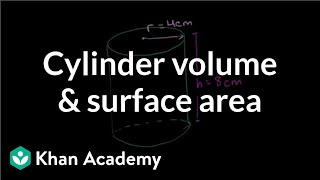By Khan Academy

The other frequently occurring shape is the cylinder. This video will introduce the cylinder and teach you the formulas for finding its surface area and volume.# Volume of a sphere | Perimeter, area, and volume | Geometry | Khan Academy

##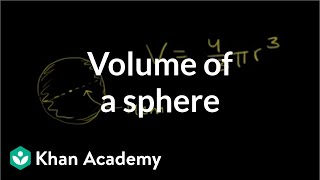By Khan Academy

"Find the volume of a sphere with a diameter of 14cm." Sal Khan solves this problem during this video clip.# Geometry - 19 - Pyramids - Volume, Lateral Area, and Total Area

##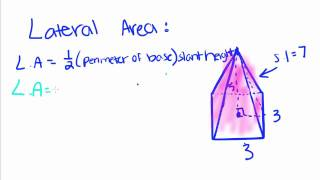By thenewboston

This video shows how to find the volume, lateral area, and total surface area of a pyramid.# Labeling parts of a circle | Perimeter, area, and volume | Geometry | Khan Academy

##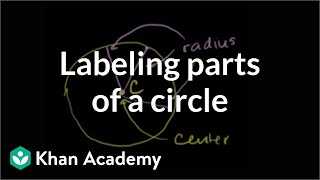By Khan Academy

Learn more: http://www.khanacademy.org/video?v=04N79tItPEA u07_l2_t3_we1 Parts of a Circle Content provided by TheNROCproject.org - (c) Monterey Institute for Technology and Education